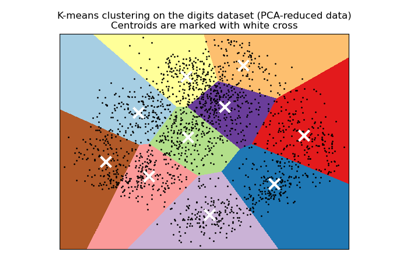# sklearn.preprocessing.scale¶

sklearn.preprocessing.scale(X, *, axis=0, with_mean=True, with_std=True, copy=True)[source]

Standardize a dataset along any axis

Center to the mean and component wise scale to unit variance.

Read more in the User Guide.

Parameters
X{array-like, sparse matrix} of shape (n_samples, n_features)

The data to center and scale.

axisint, default=0

axis used to compute the means and standard deviations along. If 0, independently standardize each feature, otherwise (if 1) standardize each sample.

with_meanbool, default=True

If True, center the data before scaling.

with_stdbool, default=True

If True, scale the data to unit variance (or equivalently, unit standard deviation).

copybool, default=True

set to False to perform inplace row normalization and avoid a copy (if the input is already a numpy array or a scipy.sparse CSC matrix and if axis is 1).

Returns
X_tr{ndarray, sparse matrix} of shape (n_samples, n_features)

The transformed data.

StandardScaler

Performs scaling to unit variance using theTransformer API (e.g. as part of a preprocessing Pipeline).

Notes

This implementation will refuse to center scipy.sparse matrices since it would make them non-sparse and would potentially crash the program with memory exhaustion problems.

Instead the caller is expected to either set explicitly with_mean=False (in that case, only variance scaling will be performed on the features of the CSC matrix) or to call X.toarray() if he/she expects the materialized dense array to fit in memory.

To avoid memory copy the caller should pass a CSC matrix.

NaNs are treated as missing values: disregarded to compute the statistics, and maintained during the data transformation.

We use a biased estimator for the standard deviation, equivalent to numpy.std(x, ddof=0). Note that the choice of ddof is unlikely to affect model performance.

For a comparison of the different scalers, transformers, and normalizers, see examples/preprocessing/plot_all_scaling.py.

Warning

Risk of data leak

Do not use scale unless you know what you are doing. A common mistake is to apply it to the entire data before splitting into training and test sets. This will bias the model evaluation because information would have leaked from the test set to the training set. In general, we recommend using StandardScaler within a Pipeline in order to prevent most risks of data leaking: pipe = make_pipeline(StandardScaler(), LogisticRegression()).

## Examples using sklearn.preprocessing.scale¶